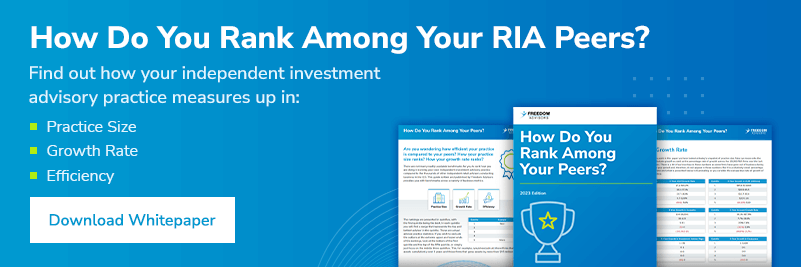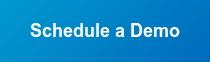In 1952 Harry Markowitz, who would later win the Nobel Prize in Economics, wrote his dissertation Portfolio Selection that continues through today to drive asset allocation. Markowitz popularized the idea, now known as modern portfolio theory (MPT), of viewing a portfolio holistically rather than at the individual security level. By viewing the sum of the parts, a person can construct an optimized portfolio that maximizes return for any given level of risk.

In MPT there are two main ideas that drive allocations: the first is that every rational investor will seek to maximize return for their given level of risk. The second is that overall portfolio risk can be reduced by adding individual investments that are unrelated or negatively related to one another.

This means that given the choice of investment A or B, with both having expected returns of 5.0% for the next 12 months, but the risk associated with investment A is twice as great as investment B, a rational investor will choose investment B.

Mean-variance analysis, commonly associated with MPT, is a process of risk-weighting a portfolio. In it, you look at the expected returns of the security and the average amount by which it varies, called variance, which can be referred to as risk. By combining investments with differing return profiles and variances, you can optimize the total return of the proposed portfolio to match the risk tolerance. Expected return is a simple weighted calculation of the percent of funds allocated to a security multiplied by the security’s expected return. To find the variance (or standard deviation) of a simple, two-asset portfolio, you need to know the covariance (correlation) between the two assets returns. The standard deviation is calculated by taking the square root of the resulting formula below:Markowitz went on to further refine risk into two separate categories: systematic risk and unsystematic risk. Systematic risk cannot be diversified away and includes risk that negatively impacts the entire market and economy. Unsystematic or idiosyncratic risk is company specific risk that can be reduced through diversification. Diversifiable risk from a specific company can be reduced by adding other assets that are not perfectly correlated. If two assets have a correlation less than one, then the combination will help to reduce the overall risk. Note from the above measure of portfolio variance, that the lower the value of ρ is from one, the more the portfolio’s risk reduces. In other words, when assets are negatively correlated or uncorrelated, it greatly reduces the overall risk profile of the portfolio.

The efficient frontier is often used as a graphical representation to show all the available combinations of securities and subsequent portfolios. The rational investor will choose for their given level of risk the appropriate portfolio falling along the efficient frontier that will maximize return. While there are many portfolios that fall below the frontier, an investor should not choose one of those options as they are sub-optimal due to insufficient return for the given risk or too much risk given the level of return.The application of modern portfolio theory and portfolio construction for an individual will need to take into account several considerations when finding an optimal combination of assets. An investor should evaluate time horizon, expected sources of return, defining assets expected returns, calculating assets covariances, expected return risk ratios, and many other considerations.

It is important to note some of the drawbacks to MPT. One is that volatility is hard to predict and another is that correlations between assets are not constant over time. During periods of financial crisis, such as the 2008-2009 Great Financial Crisis and the pandemic-induced crash in March-April 2020, assets became highly correlated to each other as most fell significantly in value at the same time. While securities can have low, zero, or even negative correlation over longer time frames, when markets experience dramatic swings variances can become highly correlated as security prices across all asset classes fall together.

Modern portfolio theory is not perfect, but it does provide a strong framework for developing a well-diversified portfolio for most investors. The ability to rebalance allocations and update security risk, return, and correlation profiles will help minimize the impacts on portfolios when markets experience heightened volatility.Topics: Portfolio Planning